Tamil Nadu Board of Secondary EducationTamil Nadu Primary School Class 4th
Advertisement Remove all ads

# Samacheer Kalvi solutions for Mathematics Term 2 - Class 4th Tamil Nadu State Board chapter 1 - Geometry [Latest edition]

Advertisement Remove all ads

#### Chapters## Chapter 1: Geometry

Exercise 1.1Exercise 1.2Exercise 1.3
Exercise 1.1 [Page 4]

### Samacheer Kalvi solutions for Mathematics Term 2 - Class 4th Tamil Nadu State Board Chapter 1 Geometry Exercise 1.1 [Page 4]

Exercise 1.1 | Q 1 | Page 4

Draw the mirror image: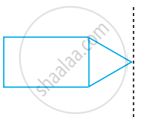Exercise 1.1 | Q 2 | Page 4

Draw the mirror image:Exercise 1.1 | Q 3 | Page 4

Draw the mirror image: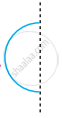Exercise 1.1 | Q 4 | Page 4

Draw the mirror image:Exercise 1.1 | Q 5 | Page 4

Draw the mirror image: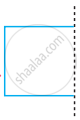Advertisement Remove all ads
Exercise 1.2 [Page 5]

### Samacheer Kalvi solutions for Mathematics Term 2 - Class 4th Tamil Nadu State Board Chapter 1 Geometry Exercise 1.2 [Page 5]

Exercise 1.2 | Q A. 1. | Page 5

Draw the line of symmetry in the following figure.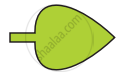Exercise 1.2 | Q A. 2. | Page 5

Draw the line of symmetry in the following figure.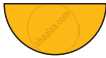Exercise 1.2 | Q A. 3. | Page 5

Draw the lines of symmetry in the following figure.Exercise 1.2 | Q A. 4. | Page 5

Draw the lines of symmetry in the following figure.Exercise 1.2 | Q B. 1. | Page 5

How many lines of symmetry for the following figureExercise 1.2 | Q B. 2. | Page 5

How many lines of symmetry for the following figure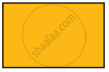Exercise 1.2 | Q B. 3. | Page 5

How many lines of symmetry for the following figureExercise 1.2 | Q B. 4. | Page 5

How many lines of symmetry for the following figureAdvertisement Remove all ads
Exercise 1.3 [Page 6]

### Samacheer Kalvi solutions for Mathematics Term 2 - Class 4th Tamil Nadu State Board Chapter 1 Geometry Exercise 1.3 [Page 6]

Exercise 1.3 | Q A. 1. | Page 6

Identify the following figure is symmetrical about dotted line?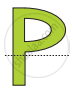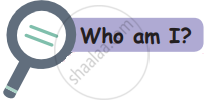I have no side, no vertices and many lines of symmetry. Who am I? ______________________

Exercise 1.3 | Q A. 2. | Page 6

Identify the following figure is symmetrical about dotted line?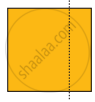I have 4 equal sides and 4 lines of symmetry. Who am I? ______________________

Exercise 1.3 | Q A. 3. | Page 6

Identify the following figure is symmetrical about dotted line?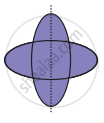I have 3 equal sides, 3 vertices and 3 lines of symmetry, who am I? _____________________

Exercise 1.3 | Q A. 4. | Page 6

Identify the following figure is symmetrical about dotted line?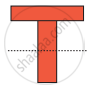I have 4 sides opposite sides are equal and I have 2 lines of symmetry. Who am I? _________________

Exercise 1.3 | Q A. 5. | Page 6

Identify the following figure is symmetrical about dotted line?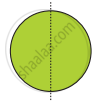Advertisement Remove all ads

## Chapter 1: Geometry

Exercise 1.1Exercise 1.2Exercise 1.3## Samacheer Kalvi solutions for Mathematics Term 2 - Class 4th Tamil Nadu State Board chapter 1 - Geometry

Samacheer Kalvi solutions for Mathematics Term 2 - Class 4th Tamil Nadu State Board chapter 1 (Geometry) include all questions with solution and detail explanation. This will clear students doubts about any question and improve application skills while preparing for board exams. The detailed, step-by-step solutions will help you understand the concepts better and clear your confusions, if any. Shaalaa.com has the Tamil Nadu Board of Secondary Education Mathematics Term 2 - Class 4th Tamil Nadu State Board solutions in a manner that help students grasp basic concepts better and faster.

Further, we at Shaalaa.com provide such solutions so that students can prepare for written exams. Samacheer Kalvi textbook solutions can be a core help for self-study and acts as a perfect self-help guidance for students.

Concepts covered in Mathematics Term 2 - Class 4th Tamil Nadu State Board chapter 1 Geometry are Concept of Symmetry, Lines of Symmetry for Regular Polygons, Concept of Reflection Symmetry, Viewing Different Sections of a Solid.

Using Samacheer Kalvi Class 4th solutions Geometry exercise by students are an easy way to prepare for the exams, as they involve solutions arranged chapter-wise also page wise. The questions involved in Samacheer Kalvi Solutions are important questions that can be asked in the final exam. Maximum students of Tamil Nadu Board of Secondary Education Class 4th prefer Samacheer Kalvi Textbook Solutions to score more in exam.

Get the free view of chapter 1 Geometry Class 4th extra questions for Mathematics Term 2 - Class 4th Tamil Nadu State Board and can use Shaalaa.com to keep it handy for your exam preparation

Advertisement Remove all ads
Share
Notifications

View all notifications

Forgot password?